## A 5.00-kg object is initially at rest. The object is acted on by a 9.00-N force toward the east for 3.00 s. No force acts on the object for

Question

A 5.00-kg object is initially at rest. The object is acted on by a 9.00-N force toward the east for 3.00 s. No force acts on the object for the next 4.00 s. How far has the object moved during this 7.00 s interval?

in progress 0
2 months 2021-07-24T00:24:44+00:00 1 Answers 16 views 0

The total distance at 7 s is: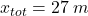Explanation:

Distance due to the force

We can use second Newton’s law to find the acceleration.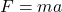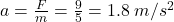Now, using the kinematic equation we will find the distance during this interval of time. Let’s recall that the initial velocity is zero.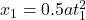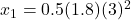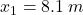In the second part of the motion, the object moves at a constant velocity, as long as there is no friction between the object and the floor.

First, we need to find the final velocity of the first interval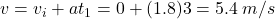So the second distance will be: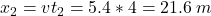Therefore, the total distance is: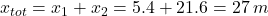I hope it helps you!